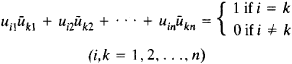# Unitary Matrix

(redirected from Unitary matrices)
Also found in: Dictionary.

## unitary matrix

[′yü·nə‚ter·ē ′mā·triks]
(mathematics)
A matrix whose inverse is equal to the complex conjugate of its transpose.
McGraw-Hill Dictionary of Scientific & Technical Terms, 6E, Copyright © 2003 by The McGraw-Hill Companies, Inc.
The following article is from The Great Soviet Encyclopedia (1979). It might be outdated or ideologically biased.

## Unitary Matrix

A unitary matrix of order n is an n × n matrix [uik] with complex entries such that the product of [uik] and its conjugate transpose [ūki] is the identity matrix E. The elements of a unitary matrix satisfy the relationsThe unitary matrices of order n form a group under multiplication. A unitary matrix with real entries is an orthogonal matrix.

References in periodicals archive ?
Moving between symmetric and unitary matrices using Cayley transformations is not a new idea.
The capacity-achieving space time modulation signal distribution at high SNR is modelled as a set of unitary matrices : {X} = [square root of T] [{[[PHI].sub.b]}.sup.B.sub.b=1] in which each matrix satisfies [[PHI].sup.*.sub.b][[PHI].sub.b] = [I.sub.M] and all [[PHI].sub.b]'s are points on a Stiefel manifold, or the subspace [[OMEGA].sub.b] spanned by column vectors of T x M matrix is uniformly distribution in Grassmann manifold [G.sub.T,M]; that is, e [G.sub.T,M] .
where ([U.sub.i,1], [U.sub.i,2]) [member of] [C.sup.NxN] and ([[LAMBDA].sub.i,1], [[LAMBDA].sub.i,2]) [member of] [C.sup.MxM] are unitary matrices. [[SIGMA].sub.i,1] = [[diag([[lambda].sub.l,l], ..., [[lambda].sub.m,1]) [0.sub.(N-M)xM]].sup.T] [member of] [C.sup.NxM] and [[SIGMA].sub.i,2] = [[diag([[lambda].sub.1,2], ..., [[lambda].sub.m,2]) [0.sub.(N-M)xM]].sup.T] [member of] [C.sup.NxM] are eigen value matrices, where [[lambda].sub.i,1] is the element of eigenvalues.
Then [P.sub.t], [Q.sub.t] are unitary matrices. The real presentation has the following properties, which are given by Jiang and Wei .
By SVD, the channel matrix, H, is decomposed into a diagonal matrix, [SIGMA], and two unitary matrices, U and V, as
Matrices [MATHEMATICAL EXPRESSION NOT REPRODUCIBLE IN ASCII] and [MATHEMATICAL EXPRESSION NOT REPRODUCIBLE IN ASCII] are unitary matrices satisfying
Yayli, Dual unitary matrices and unit dual quaternions, Differential Geometry Dynamical Systems, 10(2008), 1-12.
U and V are real N x N unitary matrices with small singular values.
Note that the technique used in the proof above can be extended easily to handle bigger input alphabets by using the matrices defined on Page 169 of [Paz71], and the method of simulating stochastic matrices by unitary matrices described in [YS09b, YS10].
This third edition features new chapters on generalized eigenvectors and numerical techniques, as well as a new section on Hermitian symmetric and unitary matrices. It offers additional exercises consisting of application, numerical, and conceptual questions.
Being subgroups of SU[(2).sub.L] x U[(1).sub.Y], they have group operations represented by 2 x 2 unitary matrices or, equivalently, by unit quaternions.

Site: Follow: Share:
Open / Close